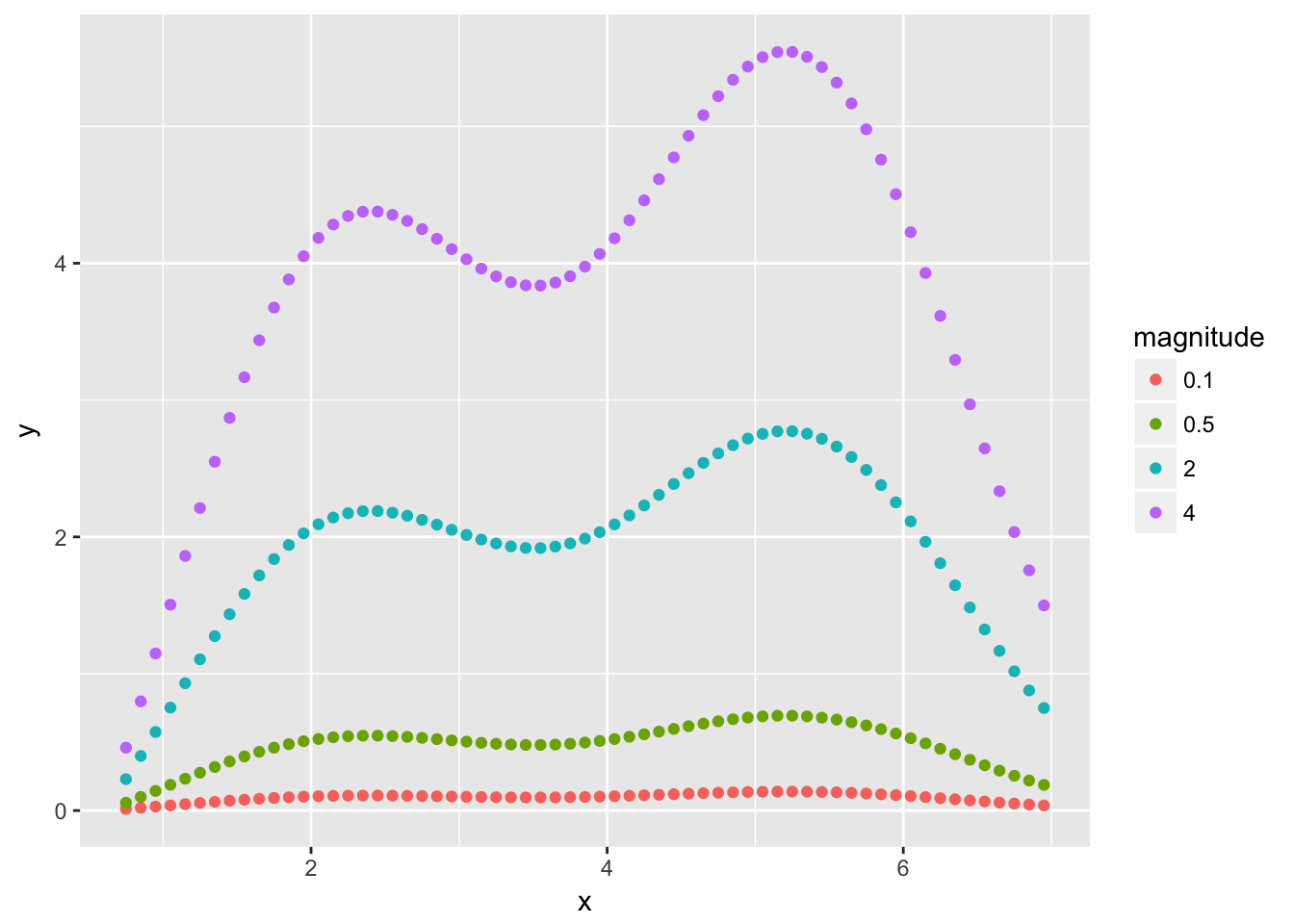citation: TBA

# Retention Time Correction with R

### Useful Libraries

``````### easy-read syntax and data-manipulation ###
library(magrittr)
library(dplyr)
library(purrr)

### Data import ###

### splines ###
library(gam)

### plotting ###
library(ggplot2)

### xml file manipulation ###
library(rvest)
library(xml2)``````

# Sample Data Generation

For demonstration, we will generate a dataset of random values that will be representative of the original referent dataset that was used to develop the method. Specifically, the generated dataset will represent a subset of measurements from the larger referent dataset.

``````nPlateWells <- 6 # Number of samples
nMetabolites <- 60 # Number of landmark metabolites per sample

# max and min values over which retention time was measures
rtMin <- 0.75
rtMax <- 7

# parameters controlling the shape and noise of the necessary corrections
diffRange <-
0.25

diffNoise <-
0.05

# We will generate samples that deviate from the target according to the function specified here.
diffShape <- function(x, magnitude) {
#sign <- ifelse(rbernoulli(1), 1, -1)
#magnitude <- runif(0.5, 2)
shape <-
(sin((x - rtMin) * pi / rtMax) + 0.5 * sin(2 * (x - rtMin) * pi / rtMax + 0.5) ^ 2)

# sign * magnitude * shape
magnitude * shape
}``````

Here, we plot examples of deviation from the target that will be generated.

``````xVals <-
seq(rtMin, rtMax, by = 0.1)

data_frame(x = rep(xVals, each = 4),
magnitude = rep(c(0.1, 0.5, 2, 4), times = length(xVals))
) %>%
group_by(magnitude) %>%
mutate(y = diffShape(x, unique(magnitude))) %>%
ungroup() %>%
mutate(magnitude = as.factor(magnitude)) %>%
ggplot() +
geom_point(aes(x = x, y = y, colour = magnitude))``````As shown, deviation from the target follows curvilinear pattern, and this is similar to the pattern of deviations observed in the original referent data.

``````# Control the shape of the deviations
shapeMagnitudes <-
seq(from = -diffRange, to = diffRange, length.out = nPlateWells + 1) %>%

# Generate unqiue metabolite IDs
metaboliteID <- runif(nMetabolites, 100, 900)
while (length(unique(metaboliteID)) < nMetabolites) {
metaboliteID <- runif(nMetabolites, 100, 900)
}

plateWellID <- seq_len(nPlateWells)

modelData <-
expand.grid(metaboliteID, plateWellID) %>%
as_data_frame() %>%
setNames(c('metaboliteID', 'plateWellID')) %>%
mutate_all(funs(as.factor))

modelData %<>%
group_by(metaboliteID) %>%
mutate(retentionTimeMean = runif(1, rtMin, rtMax),
diffShape = diffShape(retentionTimeMean, shapeMagnitudes),
diffNoise = rnorm(n(), sd = diffNoise)) %>% # Add noise to the shape of the differences
ungroup()

modelData %<>%
mutate(diff = diffShape + diffNoise,
retentionTime = retentionTimeMean + diff) %>%
# mutate(retentionTime = abs(retentionTime)) %>%
select(-diffShape, -diffNoise) %>%
arrange(plateWellID, metaboliteID)

modelData``````
``````## # A tibble: 360 × 5
##        metaboliteID plateWellID retentionTimeMean       diff retentionTime
##              <fctr>      <fctr>             <dbl>      <dbl>         <dbl>
## 1  104.635294526815           1          4.285728 -0.2737884      4.011940
## 2  108.513828553259           1          5.820129 -0.2922628      5.527866
## 3  133.057207241654           1          5.969376 -0.2560011      5.713375
## 4  135.811735503376           1          4.883059 -0.3479327      4.535126
## 5  136.211075261235           1          4.556626 -0.2947667      4.261859
## 6   151.01589281112           1          5.957611 -0.2354128      5.722198
## 7  162.784640304744           1          3.918572 -0.2844270      3.634145
## 8  180.653134919703           1          6.107188 -0.2857304      5.821457
## 9  204.986423254013           1          2.136720 -0.2703281      1.866392
## 10 212.333015166223           1          5.945104 -0.2777386      5.667366
## # ... with 350 more rows``````

For our sample data, the columns are:

• metaboliteID
• Numeric ID representing a unique measured eicosanoid metabolite.
• plateWellID
• Numeric ID representing a sample in which metabolites were measured.
• retentionTimeMean
• The mean retention time of metabolite across all samples. For our dataset, the mean is assumed to be calculated from the whole dataset, of which our sample data is a subset. The mean is the referent value that we attempt to adjust values to.
• retentionTime
• The measured retention time of a metabolite in a given sample.
• diff
• The difference between the referent value and the measured value. These differences are what we attempt to model as a function of retention time.
``````modelData %>%
ggplot(aes(x = retentionTimeMean, y = diff, colour = plateWellID)) +
geom_point() +
scale_color_discrete(guide = FALSE) +
stat_smooth(colour = 'black') +
facet_wrap(~ plateWellID) +
ggtitle('Difference from Referent for 6 Samples') +
ylab('Difference from Referent (minutes)') +
xlab('Referent Retention Time (minutes)')``````